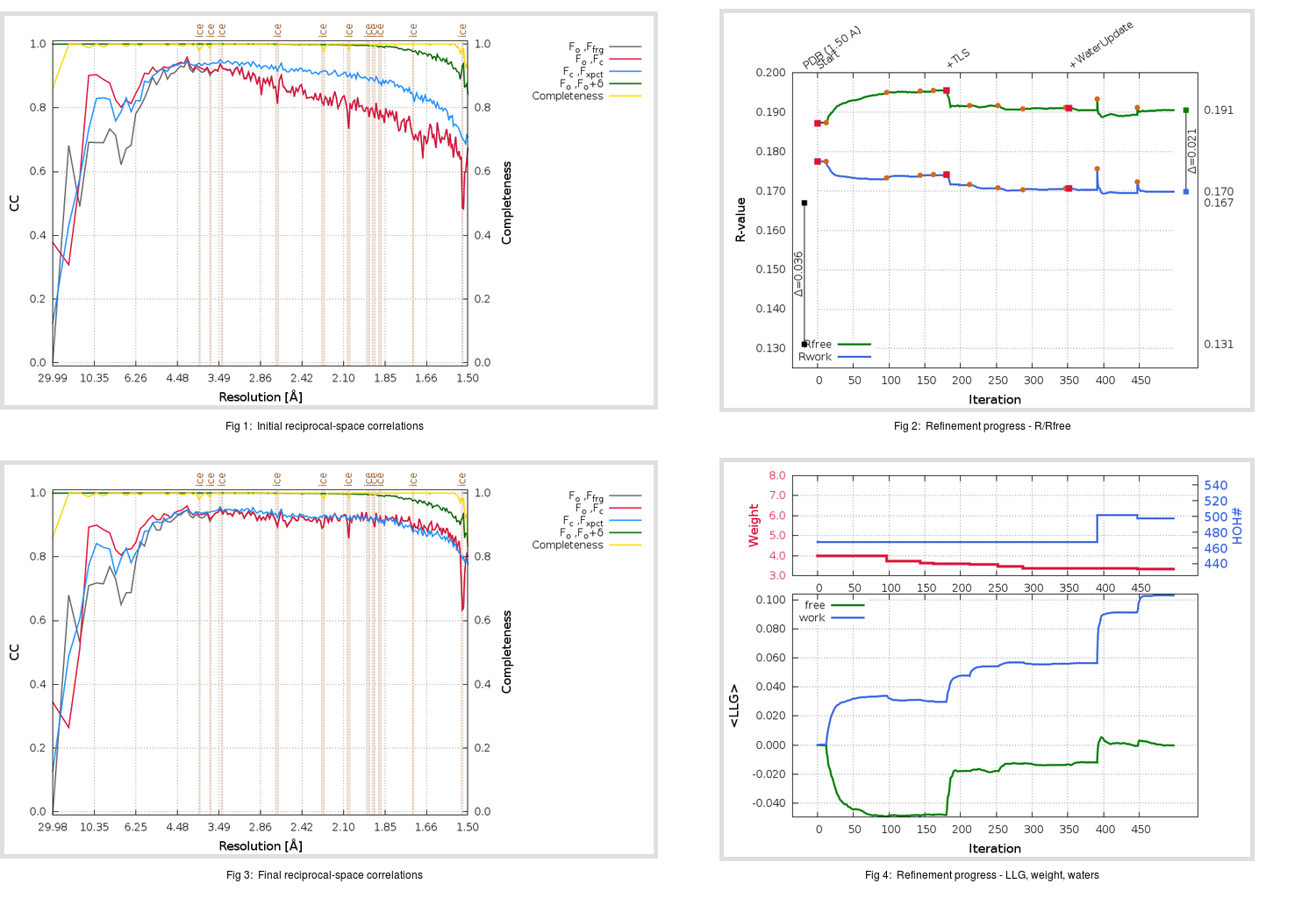Content:

```    Diffraction limits & principal axes of ellipsoid fitted to diffraction cut-off surface:
1.597         1.0000   0.0000   0.0000       a*
1.471         0.0000   1.0000   0.0000       b*
1.442         0.0000   0.0000   1.0000       c*
```

## Deposited

` `
 Date deposited Date data collection Resolution R, Rfree 20181206 20180523 1.50 0.1290 0.1670

Molprobity (CCP4 7.0 version) summary:

```Ramachandran outliers =   0.00 %
favored =  98.43 %
Rotamer outliers      =   0.93 %
C-beta deviations     =     0
Clashscore            =   2.97
RMS(bonds)            =   0.0121
RMS(angles)           =   1.72
MolProbity score      =   1.09
Resolution            =   1.50
R-work                =   0.1290
R-free                =   0.1670
```

```Number of waters      =   468

<B> (all atoms) =   21.86 ( sd =   10.39 ) for       4206 non-hydrogen atoms
<B>   (protein) =   19.96 ( sd =    8.68 ) for       3738 non-hydrogen atoms
<B>     (water) =   35.80 ( sd =   10.18 ) for        468 non-hydrogen atoms
<B>    (others) =    0.00 ( sd =    0.00 ) for          0 non-hydrogen atoms

B min/max       (all non-hydrogen atoms) =   10.07 /   76.81
B min/max   (protein non-hydrogen atoms) =   10.07 /   76.81
B min/max     (water non-hydrogen atoms) =   13.57 /   67.58
B min/max     (other non-hydrogen atoms) =    0.00 /    0.00
```

## BUSTER (re-)refinement

` `

Molprobity (CCP4 7.0 version) summary:

```Ramachandran outliers =   0.00 %
favored =  98.43 %
Rotamer outliers      =   0.70 %
C-beta deviations     =     1
Clashscore            =   2.03
RMS(bonds)            =   0.0112
RMS(angles)           =   1.59
MolProbity score      =   0.97
Resolution            =   1.50
R-work                =   0.1698
R-free                =   0.1905
```

```Number of waters      =   498

<B> (all atoms) =   21.94 ( sd =   10.03 ) for       4236 non-hydrogen atoms
<B>   (protein) =   19.89 ( sd =    7.58 ) for       3738 non-hydrogen atoms
<B>     (water) =   36.08 ( sd =   12.04 ) for        498 non-hydrogen atoms
<B>    (others) =    0.00 ( sd =    0.00 ) for          0 non-hydrogen atoms

B min/max       (all non-hydrogen atoms) =   10.21 /   86.85
B min/max   (protein non-hydrogen atoms) =   10.21 /   64.09
B min/max     (water non-hydrogen atoms) =   13.15 /   86.85
B min/max     (other non-hydrogen atoms) =    0.00 /    0.00
```

Refinement progression:Results:

` `
 File Remark 6NB8_aB_refine.01_03_refine.pdb.gz exact refinement commands are in header 6NB8_aB_refine.01_03_refine.mtz.gz including original deposited data and several re-refinement map coefficients 6NB8_aB_refine.01_03_BUSTER_model.cif.gz including any non-standard compound restraints 6NB8_aB_refine.01_03_BUSTER_refln.cif.gz